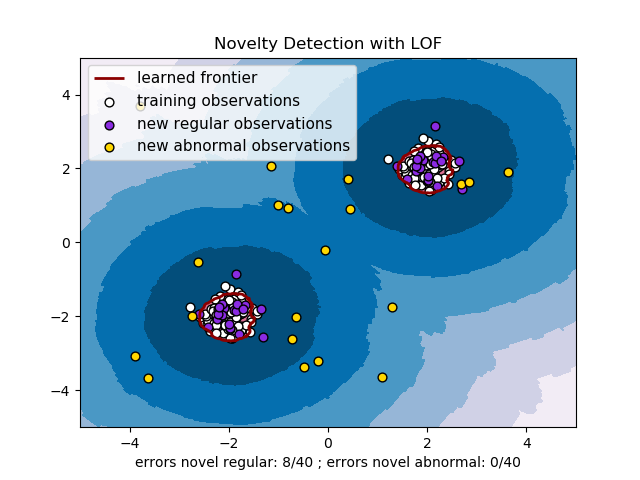# Novelty detection with Local Outlier Factor (LOF)¶

The Local Outlier Factor (LOF) algorithm is an unsupervised anomaly detection method which computes the local density deviation of a given data point with respect to its neighbors. It considers as outliers the samples that have a substantially lower density than their neighbors. This example shows how to use LOF for novelty detection. Note that when LOF is used for novelty detection you MUST not use predict, decision_function and score_samples on the training set as this would lead to wrong results. You must only use these methods on new unseen data (which are not in the training set). See User Guide: for details on the difference between outlier detection and novelty detection and how to use LOF for outlier detection.

The number of neighbors considered, (parameter n_neighbors) is typically set 1) greater than the minimum number of samples a cluster has to contain, so that other samples can be local outliers relative to this cluster, and 2) smaller than the maximum number of close by samples that can potentially be local outliers. In practice, such informations are generally not available, and taking n_neighbors=20 appears to work well in general.import numpy as np
import matplotlib
import matplotlib.pyplot as plt
from sklearn.neighbors import LocalOutlierFactor

print(__doc__)

np.random.seed(42)

xx, yy = np.meshgrid(np.linspace(-5, 5, 500), np.linspace(-5, 5, 500))
# Generate normal (not abnormal) training observations
X = 0.3 * np.random.randn(100, 2)
X_train = np.r_[X + 2, X - 2]
# Generate new normal (not abnormal) observations
X = 0.3 * np.random.randn(20, 2)
X_test = np.r_[X + 2, X - 2]
# Generate some abnormal novel observations
X_outliers = np.random.uniform(low=-4, high=4, size=(20, 2))

# fit the model for novelty detection (novelty=True)
clf = LocalOutlierFactor(n_neighbors=20, novelty=True, contamination=0.1)
clf.fit(X_train)
# DO NOT use predict, decision_function and score_samples on X_train as this
# would give wrong results but only on new unseen data (not used in X_train),
# e.g. X_test, X_outliers or the meshgrid
y_pred_test = clf.predict(X_test)
y_pred_outliers = clf.predict(X_outliers)
n_error_test = y_pred_test[y_pred_test == -1].size
n_error_outliers = y_pred_outliers[y_pred_outliers == 1].size

# plot the learned frontier, the points, and the nearest vectors to the plane
Z = clf.decision_function(np.c_[xx.ravel(), yy.ravel()])
Z = Z.reshape(xx.shape)

plt.title("Novelty Detection with LOF")
plt.contourf(xx, yy, Z, levels=np.linspace(Z.min(), 0, 7), cmap=plt.cm.PuBu)
a = plt.contour(xx, yy, Z, levels=, linewidths=2, colors='darkred')
plt.contourf(xx, yy, Z, levels=[0, Z.max()], colors='palevioletred')

s = 40
b1 = plt.scatter(X_train[:, 0], X_train[:, 1], c='white', s=s, edgecolors='k')
b2 = plt.scatter(X_test[:, 0], X_test[:, 1], c='blueviolet', s=s,
edgecolors='k')
c = plt.scatter(X_outliers[:, 0], X_outliers[:, 1], c='gold', s=s,
edgecolors='k')
plt.axis('tight')
plt.xlim((-5, 5))
plt.ylim((-5, 5))
plt.legend([a.collections, b1, b2, c],
["learned frontier", "training observations",
"new regular observations", "new abnormal observations"],
loc="upper left",
prop=matplotlib.font_manager.FontProperties(size=11))
plt.xlabel(
"errors novel regular: %d/40 ; errors novel abnormal: %d/40"
% (n_error_test, n_error_outliers))
plt.show()


Total running time of the script: ( 0 minutes 0.634 seconds)

Gallery generated by Sphinx-Gallery